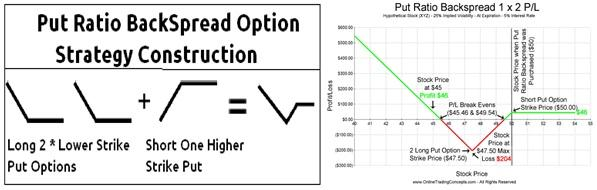Posted in Finance, Accounting and Economics Terms, Total Reads: 1000

It is an option trading strategy where one takes both short put and long put and the profit/loss of his/her position is determined by the ratio of those puts. As the option investor determines the puts ratio and he/she derives profit from the volatility of underlying asset it, the name is given as Put ratio Backspread.

The option is designed such that it has a potential to make unlimited profit with limited loss or limited profit with unlimited loss depending upon the preference of the investor. Typical ratios are (long put : Short put) 3:2, 2:1, 3:1For example: Take a stock which is treading at \$29.50. It has following one month puts:

1. Exercise price= \$30

Option price= \$1.16

2. Exercise price= \$29

Option price= 62 cents

Now an investor who is bearish on the stock would set a 2:1 put ratio stock spread:

Considering each option contract has 100 shares,

Long on two \$29 put contract= (\$.62*2*100) = (\$124)

Short on one \$30 put contract= \$1.16*100= \$116

Net cost= \$8

On expiry:

Case 1:

Stock price=27

Gain(in \$)= (29-27)*200-(30-27)*1000-8 =92

Case 2:

Stock Price=31

No one will get exercised

Loss(in \$)=8

Hence, this concludes the definition of Put Ratio Backspread along with its overview.

Browse the definition and meaning of more terms similar to Put Ratio Backspread. The Management Dictionary covers over 7000 business concepts from 6 categories.

Search & Explore : Management Dictionary

Similar Definitions from same Category: